Download Presentation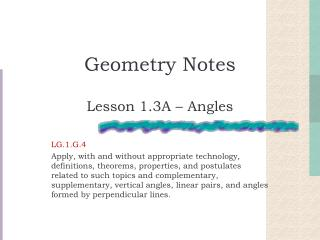Geometry Notes

# Geometry Notes - PowerPoint PPT Presentation

Geometry Notes. Lesson 1.3A – Angles LG.1.G.4 Apply, with and without appropriate technology, definitions, theorems, properties, and postulates related to such topics and complementary, supplementary, vertical angles, linear pairs, and angles formed by perpendicular lines. Angle.I am the owner, or an agent authorized to act on behalf of the owner, of the copyrighted work described.
Download Presentation## Geometry Notes

An Image/Link below is provided (as is) to download presentation

Download Policy: Content on the Website is provided to you AS IS for your information and personal use and may not be sold / licensed / shared on other websites without getting consent from its author.While downloading, if for some reason you are not able to download a presentation, the publisher may have deleted the file from their server.

- - - - - - - - - - - - - - - - - - - - - - - - - - E N D - - - - - - - - - - - - - - - - - - - - - - - - - -
Presentation Transcript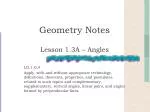### Geometry Notes

Lesson 1.3A – Angles

LG.1.G.4

Apply, with and without appropriate technology, definitions, theorems, properties, and postulates related to such topics and complementary, supplementary, vertical angles, linear pairs, and angles formed by perpendicular lines.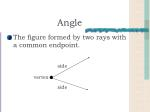Angle
• The figure formed by two rays with a common endpoint.

side

vertex

side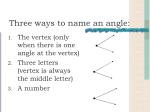Three ways to name an angle:
• The vertex (only when there is one angle at the vertex)
• Three letters (vertex is always the middle letter)
• A number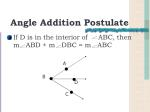A

B

D

C

• If D is in the interior of ABC, then m ABD + m DBC = m ABC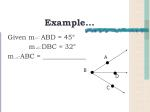A

B

D

C

Example…

Given m ABD = 45°

m DBC = 32°

m ABC = _____________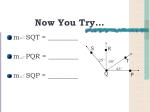S

R

25°

50°

T

43°

Q

P

Now You Try…
• m SQT = _________
• m PQR = _________
• m SQP = _________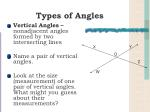X

Y

O

Z

W

Types of Angles
• Vertical Angles – nonadjacent angles formed by two intersecting lines
• Name a pair of vertical angles.
• Look at the size (measurement) of one pair of vertical angles. What might you guess about their measurements?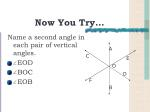A

C

E

O

D

F

B

Now You Try…

Name a second angle in each pair of vertical angles.

• EOD
• BOC
• EOB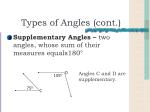D

105O

75O

C

Types of Angles (cont.)
• Supplementary Angles – two angles, whose sum of their measures equals180°

Angles C and D are supplementary.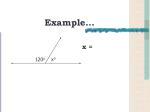xo

120o

Example…

x =50O

A

40o

B

Types of Angles (cont.)
• Complementary Angles – two angles, whose sum of their measures equals 90°

Angles A and B are complementary.K

L

P

M

N

Example . . .

Given: KLM is a right angle.

• If m KLP = 57°, find m MLP.
• If m MLP = 69°, find m KLP.
• If m NPM = 120°, find m MPL.
• If m KPL = 130°, find m KPNB

C

E

D

A

Now you try. . .

Given: BAD is a right angle.

• If m BAE = 74°, find m EAD.
• If m EAD = 36°, find m BAE.
• If m CED = 71°, find m CEB.
• If m AED = 117°, find m BEA.